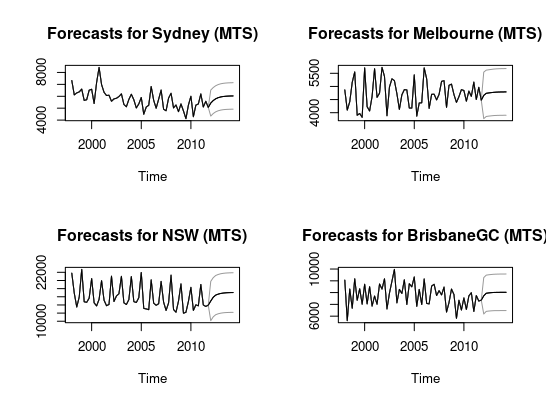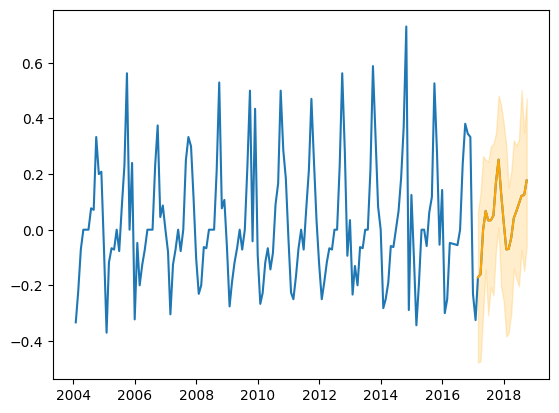This article was first published on T. Moudiki's Webpage - Python , and kindly contributed to python-bloggers. (You can report issue about the content on this page here)

Version `v0.14.0` of nnetsauce is now available for R (hopefully a rapid installation) and Python on GitHub, PyPI and conda. It’s been mainly tested on Linux and macOS. For Windows users, you can try to install of course, but if it doesn’t work, please use WSL2.

NEWS

• update and align as much as possible with `R` version (new plotting function for multivariate time series (`MTS`), `plot.MTS`, is not `S3`, but it’s complicated)
```# 0 - install packages ----------------------------------------------------

#utils::install.packages("remotes")
remotes::install_github("Techtonique/nnetsauce/R-package", force = TRUE)

# 1 - ENET simulations ----------------------------------------------------

obj <- nnetsauce::sklearn\$linear_model\$ElasticNet()
obj2 <- nnetsauce::MTS(obj,
start_input = start(fpp::vn),
frequency_input = frequency(fpp::vn),
kernel = "gaussian", replications = 100L)
X <- data.frame(fpp::vn)
obj2\$fit(X)
obj2\$predict(h = 10L)
typeof(obj2)

par(mfrow=c(2, 2))
plot.MTS(obj2, selected_series = "Sydney")
plot.MTS(obj2, selected_series = "Melbourne")
plot.MTS(obj2, selected_series = "NSW")
plot.MTS(obj2, selected_series = "BrisbaneGC")

# 2 - Bayesian Ridge ----------------------------------------------------

obj <- nnetsauce::sklearn\$linear_model\$BayesianRidge()
obj2 <- nnetsauce::MTS(obj,
start_input = start(fpp::vn),
frequency_input = frequency(fpp::vn))
X <- data.frame(fpp::vn)
obj2\$fit(X)
obj2\$predict(h = 10L, return_std = TRUE)

par(mfrow=c(2, 2))
plot.MTS(obj2, selected_series = "Sydney")
plot.MTS(obj2, selected_series = "Melbourne")
plot.MTS(obj2, selected_series = "NSW")
plot.MTS(obj2, selected_series = "BrisbaneGC")
```• colored graphics for `Python` class `MTS`
```# !pip install nnetsauce —upgrade

import nnetsauce as ns
import numpy as np
import pandas as pd
from sklearn.linear_model import Ridge, BayesianRidge
from sklearn.ensemble import RandomForestRegressor
from time import time

url = "https://raw.githubusercontent.com/thierrymoudiki/mts-data/master/heater-ice-cream/ice_cream_vs_heater.csv"

# ice cream vs heater (I don't own the copyright)
df.set_index('Month', inplace=True)
df.index.rename('date')

df = df.pct_change().dropna()

idx_train = int(df.shape*0.8)
idx_end = df.shape
df_train = df.iloc[0:idx_train,]

regr3 = Ridge()
obj_MTS3 = ns.MTS(regr3, lags = 4, n_hidden_features=7, #IRL, must be tuned
replications=50, kernel='gaussian',
seed=24, verbose = 1)
start = time()
obj_MTS3.fit(df_train)
print(f"Elapsed {time()-start} s")

obj_MTS3.plot("heater")
obj_MTS3.plot("ice cream")
```